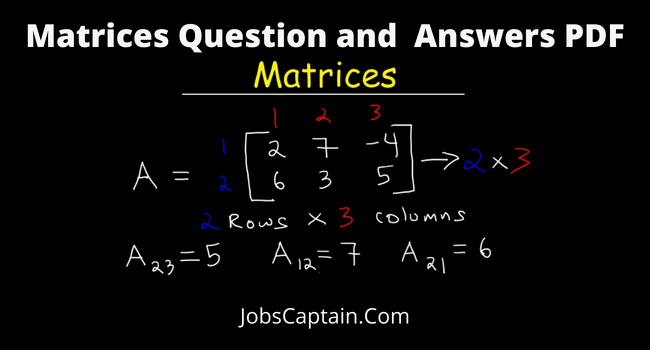# 10+ Matrices Questions and Answers PDF

By | August 22, 2022Hello Students, Today we will share with you the Matrices Questions and Answers PDF.

This Book is useful for students who are preparing for competitive exams such as all SSC Exams and Railways Exams. This will be helpful for increasing your practice and also to increase your marks in Competitive Exams. We will be also sharing the Matrices Questions and Answers PDF Class 10 and class 12.

We have provided the Multiple Choice Questions and Answers to test your knowledge about the Matrices. So try to solve every question to start your preparations now.

Let’s see the basic information on the topic:

Content Index

## MCQ Questions on Matrices:

Question 1: If A is a square matrix such that A2 = A, then (I – A)3 + A is equal to __________.

(A) I

(B) 0

(C) I – A

(D) I + A

(A) I

Question 2: Total number of possible matrices of order 3 × 3 with each entry 2 or 0 is _________.

(A) 9

(B) 27

(C) 81

(D) 512

(D) 512

Question 3: If A and B are two matrices of the order 3 × m and 3 × n, respectively, and m = n, then the order of matrix (5A – 2B) is ___________.

(A) m x 3

(B) 3 x 3

(C) m x n

(D) 3 x n

(D) 3 x n

Question 4: for any two matrices A and B, we have __________.

(A) AB = BA

(B) AB ≠ BA

(C) AB = O

(D) None of these

(D) None of these

Question 5: If A and B are symmetric matrices of the same order, then (AB′ –BA′) is a __________.

(A) Skew symmetric matrix

(B) Null matrix

(C) Symmetric matrix

(D) None of these

(A) Skew symmetric matrix

• What’s include
• a) Elements matrix
b) Row matrix
c) Column matrix
d) Diagonal matrix
e) Scalar matrix
f) Unit matrix OR Identity matrix
g) Triangular matrix
h) Comparable matrices
i) Equality of matrices
j) Skew-symmetric matrix
• Matrics Exam questions by T. Madas – View PDF
• Class 12 Matrices DGT Mathematics Study Material PDF – Download Now

Also read these maths study materials:

We will soon be posting the Matrices and Determinants Questions with Solutions. So stay connected to the website to get full updates on our posts.

Thank you for reading. Furthermore, If you have any doubt about the Matrices Questions or if you want any other MCQ Quiz then let us know by commenting in the comment section.

Category: Class 11 - 12 Science Maths PDF